# Linear Systems COS 323 Linear Systems Linear Systems

• Slides: 41Linear Systems COS 323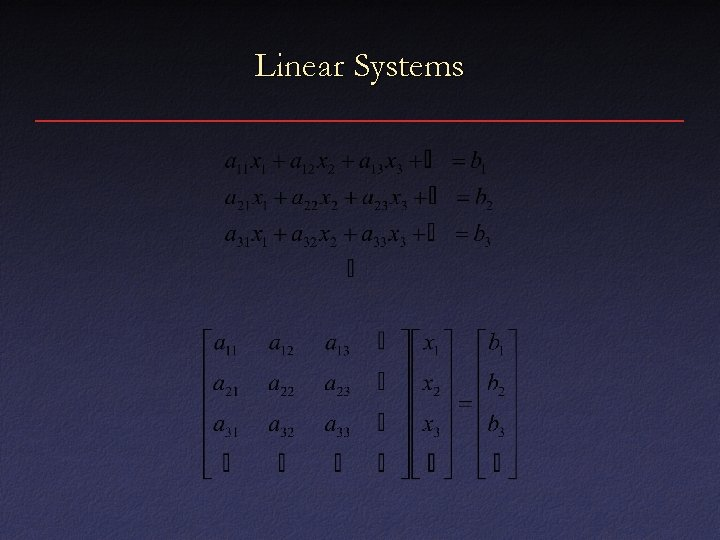Linear Systems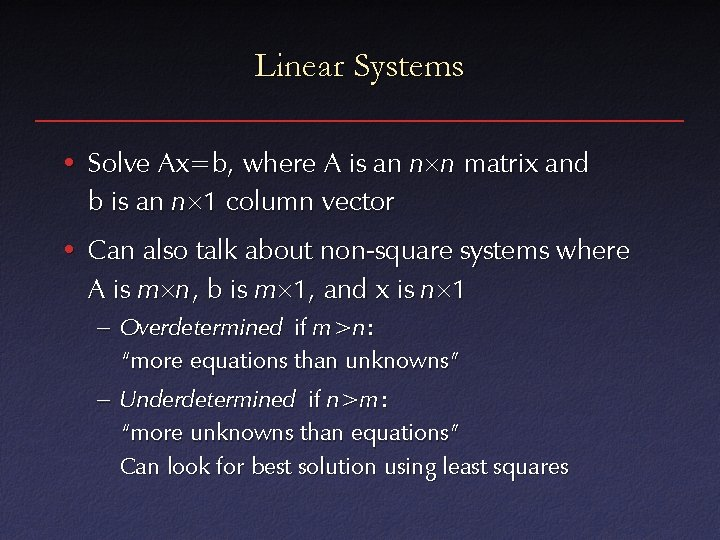Linear Systems • Solve Ax=b, where A is an n n matrix and b is an n 1 column vector • Can also talk about non-square systems where A is m n, b is m 1, and x is n 1 – Overdetermined if m>n: “more equations than unknowns” – Underdetermined if n>m: “more unknowns than equations” Can look for best solution using least squares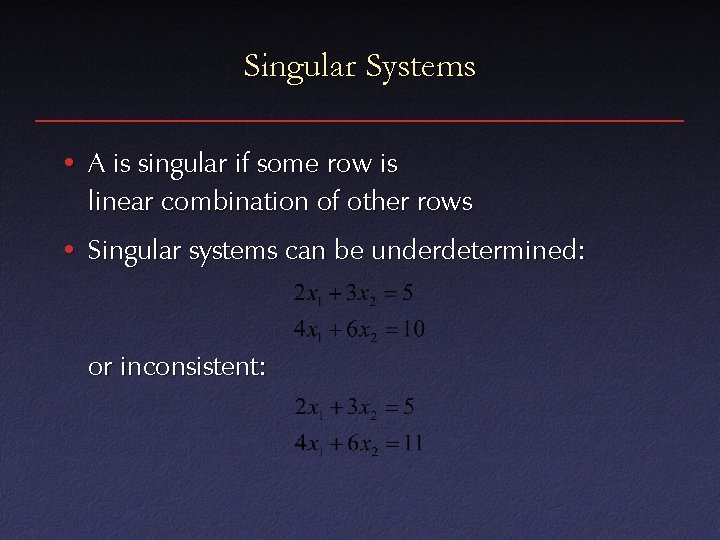Singular Systems • A is singular if some row is linear combination of other rows • Singular systems can be underdetermined: or inconsistent: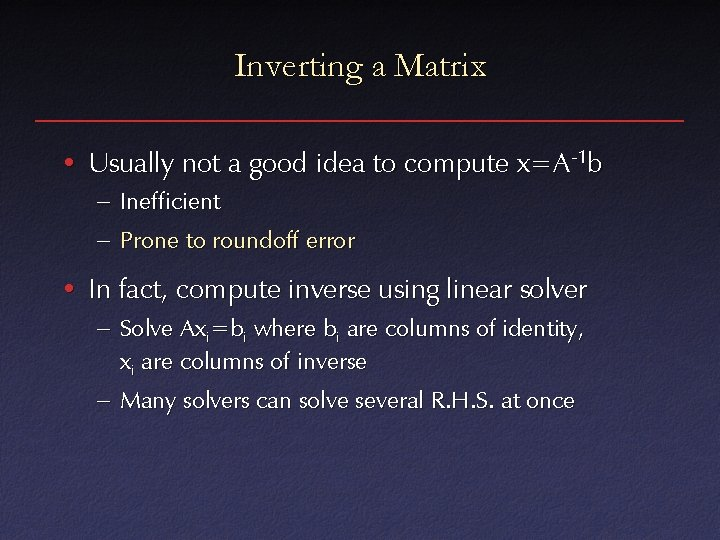Inverting a Matrix • Usually not a good idea to compute x=A-1 b – Inefficient – Prone to roundoff error • In fact, compute inverse using linear solver – Solve Axi=bi where bi are columns of identity, xi are columns of inverse – Many solvers can solve several R. H. S. at onceGauss-Jordan Elimination • Fundamental operations: 1. Replace one equation with linear combination of other equations 2. Interchange two equations 3. Re-label two variables • Combine to reduce to trivial system • Simplest variant only uses #1 operations, but get better stability by adding #2 (partial pivoting) or #2 and #3 (full pivoting)Gauss-Jordan Elimination • Solve: • Only care about numbers – form “tableau” or “augmented matrix”:Gauss-Jordan Elimination • Given: • Goal: reduce this to trivial system and read off answer from right column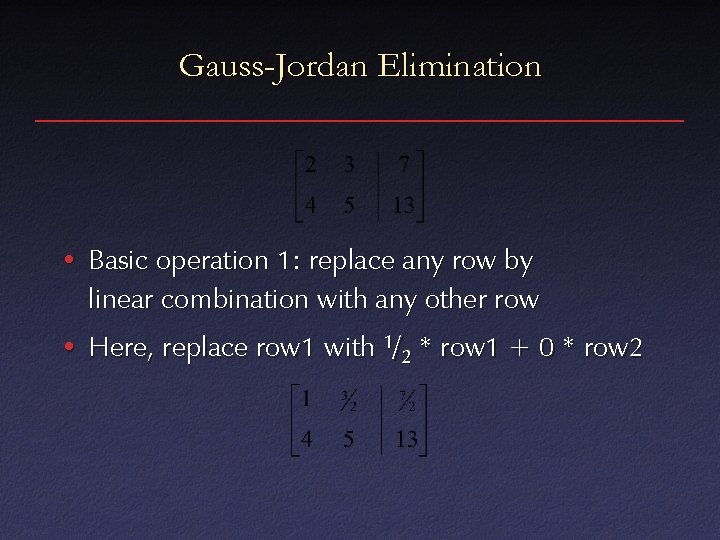Gauss-Jordan Elimination • Basic operation 1: replace any row by linear combination with any other row • Here, replace row 1 with 1/2 * row 1 + 0 * row 2Gauss-Jordan Elimination • Replace row 2 with row 2 – 4 * row 1 • Negate row 2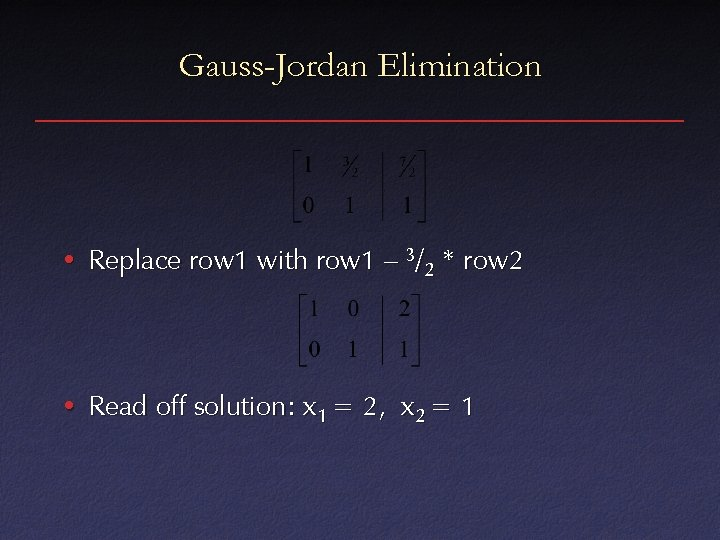Gauss-Jordan Elimination • Replace row 1 with row 1 – 3/2 * row 2 • Read off solution: x 1 = 2, x 2 = 1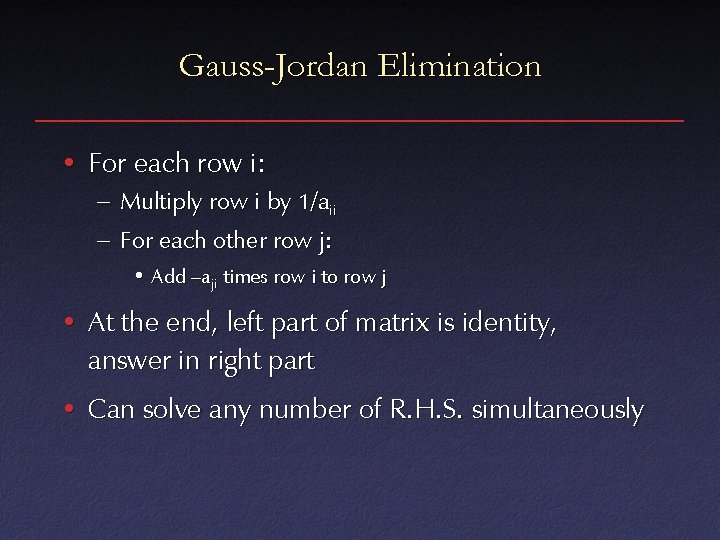Gauss-Jordan Elimination • For each row i: – Multiply row i by 1/aii – For each other row j: • Add –aji times row i to row j • At the end, left part of matrix is identity, answer in right part • Can solve any number of R. H. S. simultaneouslyPivoting • Consider this system: • Immediately run into problem: algorithm wants us to divide by zero! • More subtle version: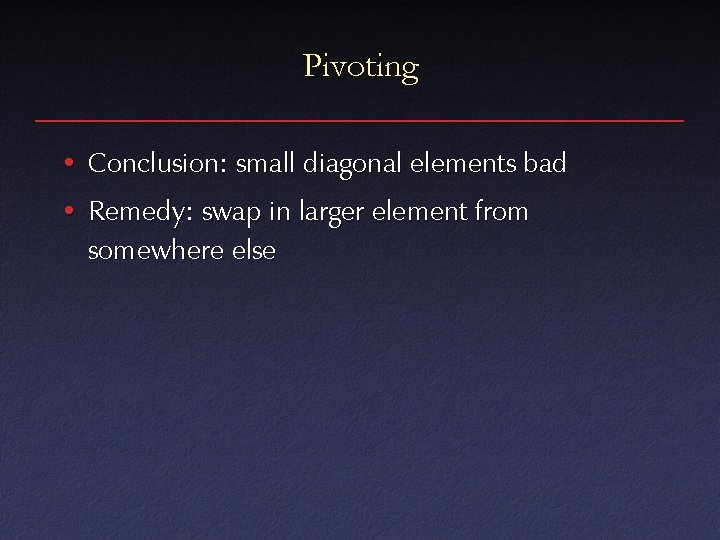Pivoting • Conclusion: small diagonal elements bad • Remedy: swap in larger element from somewhere else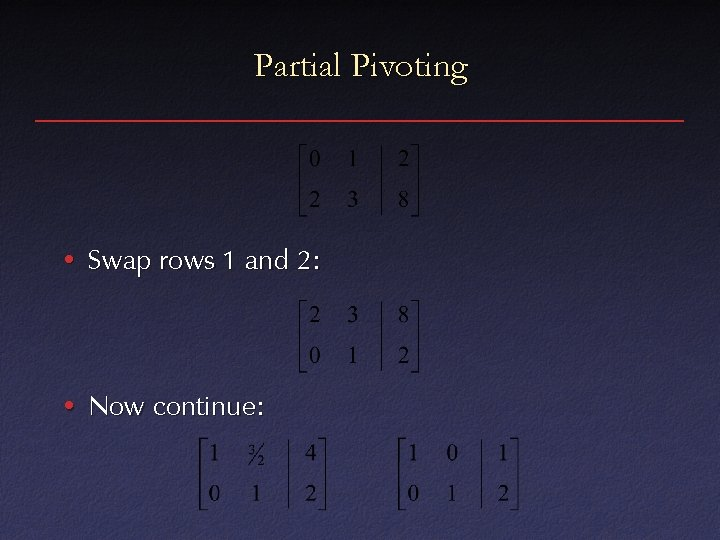Partial Pivoting • Swap rows 1 and 2: • Now continue:Full Pivoting • Swap largest element onto diagonal by swapping rows 1 and 2 and columns 1 and 2: • Critical: when swapping columns, must remember to swap results!Full Pivoting * Swap results 1 and 2 • Full pivoting more stable, but only slightly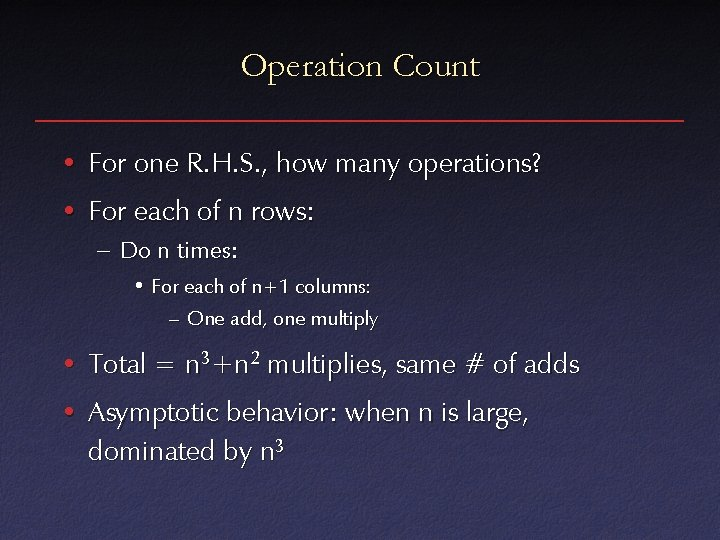Operation Count • For one R. H. S. , how many operations? • For each of n rows: – Do n times: • For each of n+1 columns: – One add, one multiply • Total = n 3+n 2 multiplies, same # of adds • Asymptotic behavior: when n is large, dominated by n 3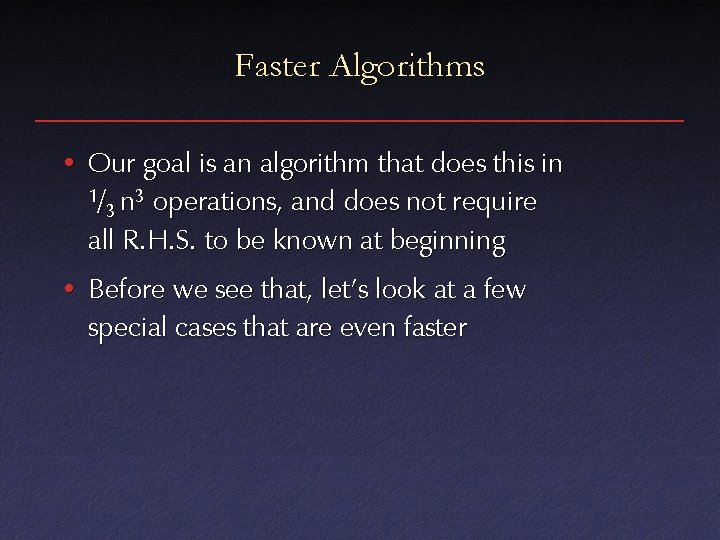Faster Algorithms • Our goal is an algorithm that does this in 1/ n 3 operations, and does not require 3 all R. H. S. to be known at beginning • Before we see that, let’s look at a few special cases that are even fasterTridiagonal Systems • Common special case: • Only main diagonal + 1 above and 1 belowSolving Tridiagonal Systems • When solving using Gauss-Jordan: – Constant # of multiplies/adds in each row – Each row only affects 2 othersRunning Time • 2 n loops, 4 multiply/adds per loop (assuming correct bookkeeping) • This running time has a fundamentally different dependence on n: linear instead of cubic – Can say that tridiagonal algorithm is O(n) while Gauss-Jordan is O(n 3)Big-O Notation • Informally, O(n 3) means that the dominant term for large n is cubic • More precisely, there exist a c and n 0 such that running time c n 3 if n > n 0 • This type of asymptotic analysis is often used to characterize different algorithms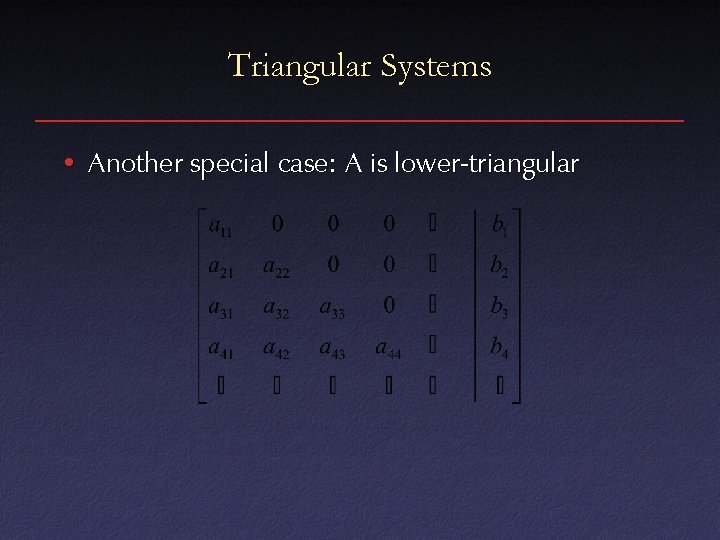Triangular Systems • Another special case: A is lower-triangularTriangular Systems • Solve by forward substitution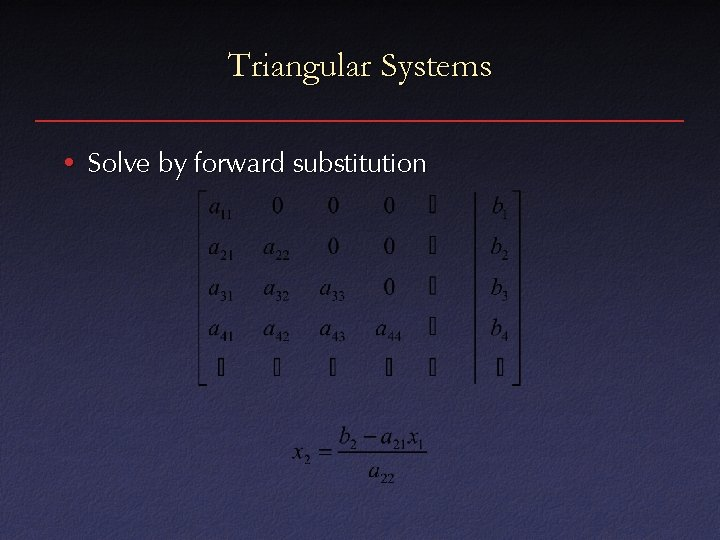Triangular Systems • Solve by forward substitution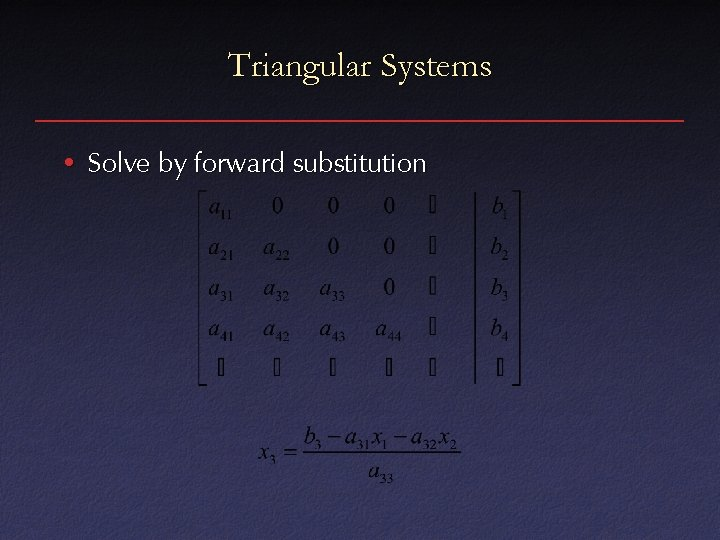Triangular Systems • Solve by forward substitutionTriangular Systems • If A is upper triangular, solve by backsubstitutionTriangular Systems • If A is upper triangular, solve by backsubstitution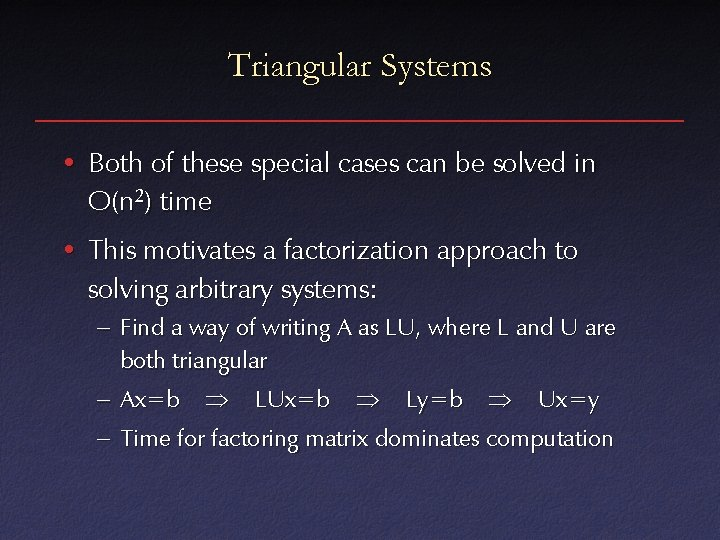Triangular Systems • Both of these special cases can be solved in O(n 2) time • This motivates a factorization approach to solving arbitrary systems: – Find a way of writing A as LU, where L and U are both triangular – Ax=b LUx=b Ly=b Ux=y – Time for factoring matrix dominates computation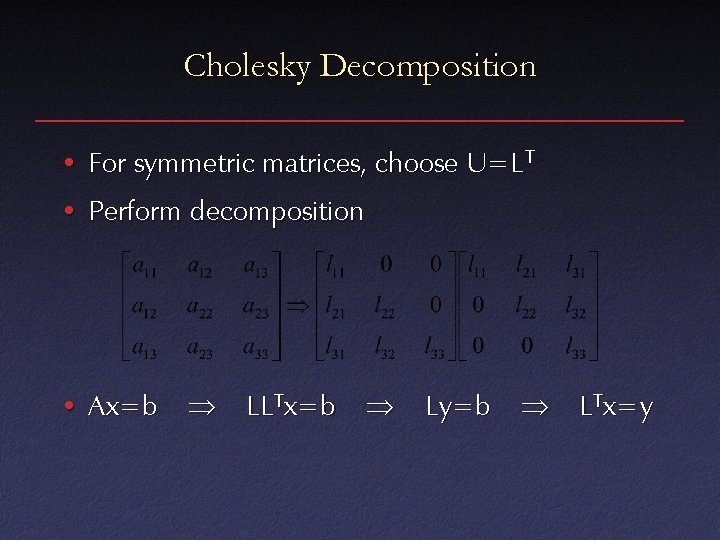Cholesky Decomposition • For symmetric matrices, choose U=LT • Perform decomposition • Ax=b LLTx=b Ly=b LTx=yCholesky DecompositionCholesky Decomposition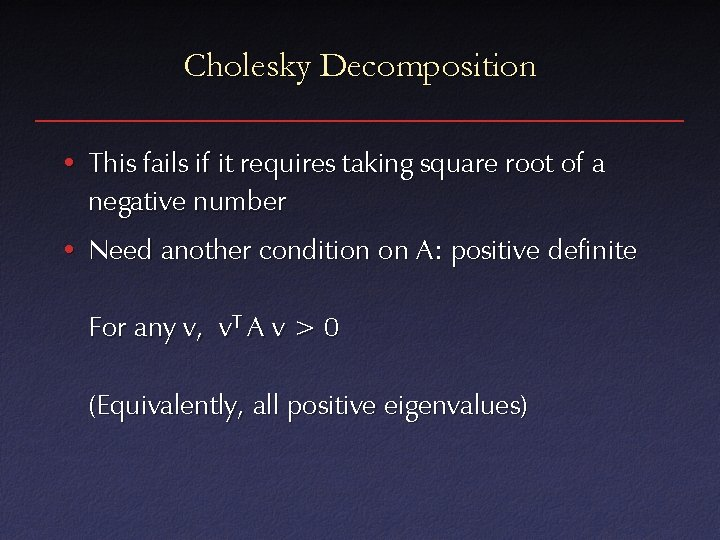Cholesky Decomposition • This fails if it requires taking square root of a negative number • Need another condition on A: positive definite For any v, v. T A v > 0 (Equivalently, all positive eigenvalues)Cholesky Decomposition • Running time turns out to be 1/6 n 3 – Still cubic, but much lower constant • Result: this is preferred method for solving symmetric positive definite systems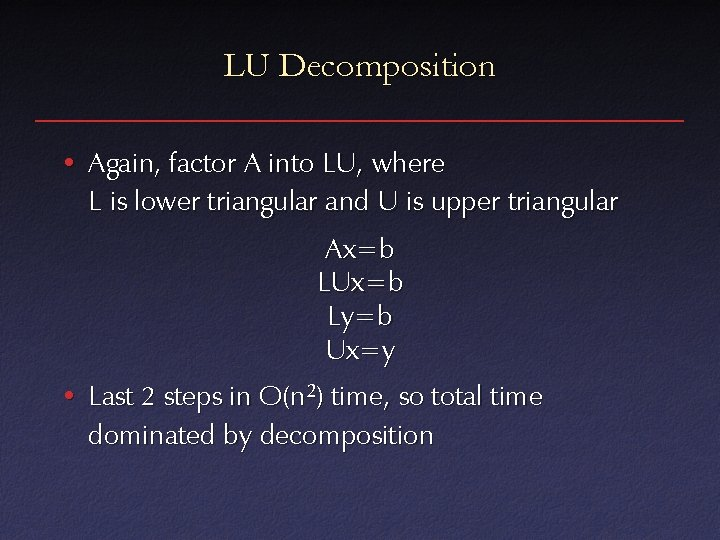LU Decomposition • Again, factor A into LU, where L is lower triangular and U is upper triangular Ax=b LUx=b Ly=b Ux=y • Last 2 steps in O(n 2) time, so total time dominated by decompositionCrout’s Method • More unknowns than equations! • Let all lii=1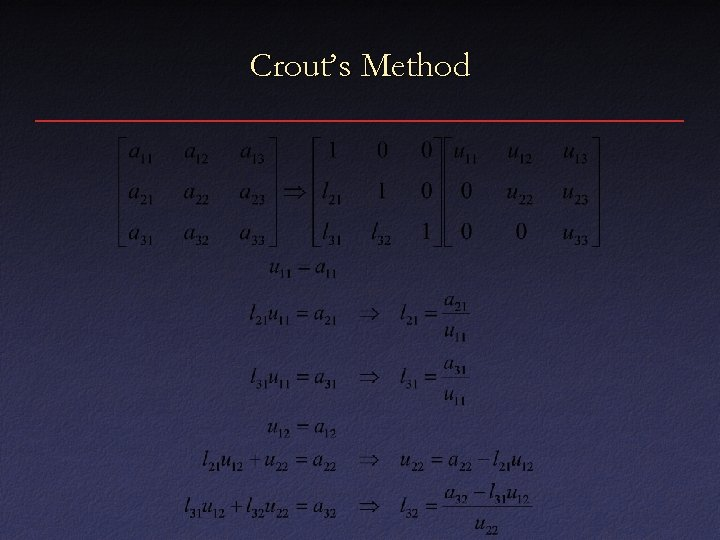Crout’s MethodCrout’s Method • For i = 1. . n – For j = 1. . i – For j = i+1. . nCrout’s Method • Interesting note: # of outputs = # of inputs, algorithm only refers to elements not output yet – Can do this in-place! – Algorithm replaces A with matrix of l and u values, 1 s are implied – Resulting matrix must be interpreted in a special way: not a regular matrix – Can rewrite forward/backsubstitution routines to use this “packed” l-u matrix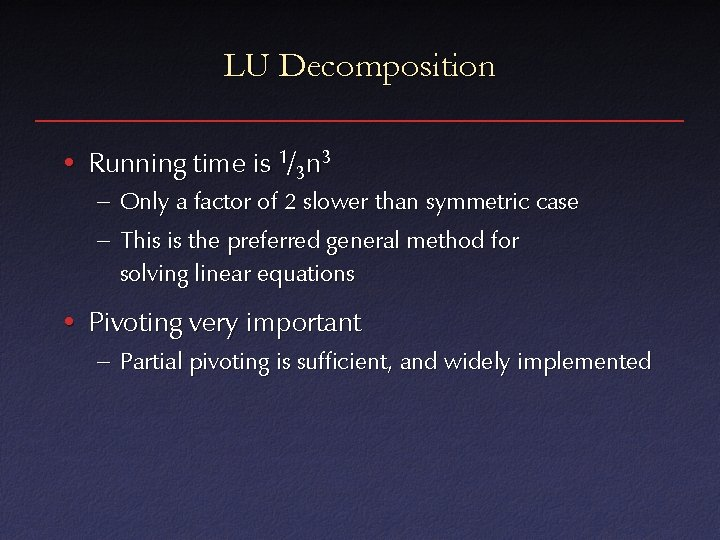LU Decomposition • Running time is 1/3 n 3 – Only a factor of 2 slower than symmetric case – This is the preferred general method for solving linear equations • Pivoting very important – Partial pivoting is sufficient, and widely implemented